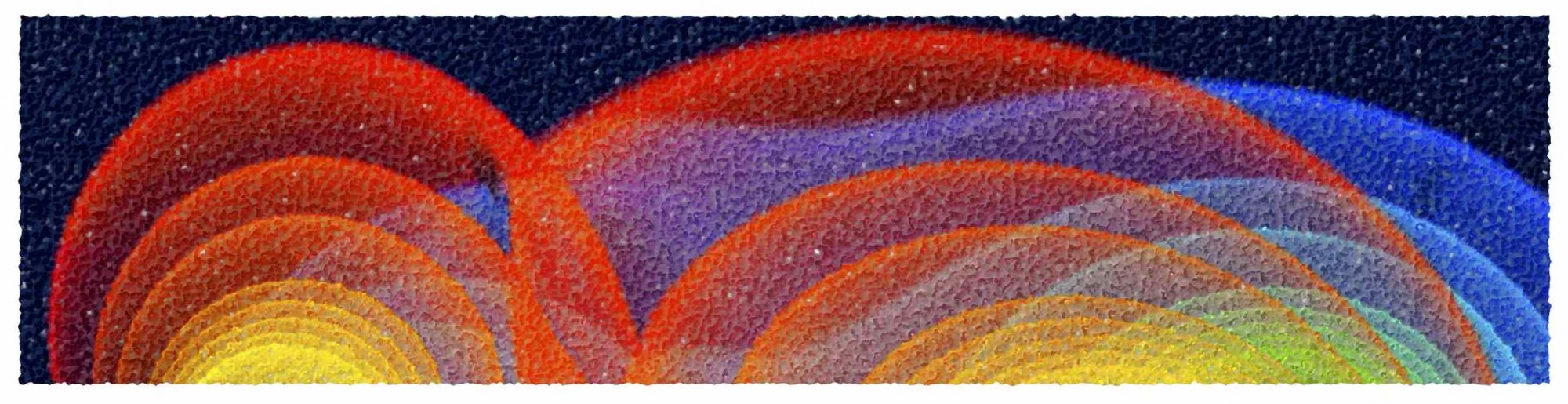# General Relativity

WS2015/2016, Wed, 09:00-11:00, Room _0.111; Thur, 08:00-10:00, Room 2.216 (exercises by Dr. Younsi)

Assigned Exercises (solutions). Scanned Lecture Notes

This is a first course to general relativity and provides an introduction to the best theory of gravity we presently know. The course also provides an introduction to the mathematical and geometrical tools needed for the formulation of the theory and ultimately for the derivation of the Einstein equations. The first part of the course will concentrate on the mathematical aspects of differential geometry and of tensor calculus. A second part of the course will provide an introduction to special relativity and to the definition of the building blocks of the theory: metric and curvature tensors. The final part of the course will concentrate on the derivation of the Einstein equations and on the study of some exact solutions. A series of compulsory exercises parallels the course. This course is propaedeutic to the “Advanced General Relativity” that will normally be offered in a following semester.